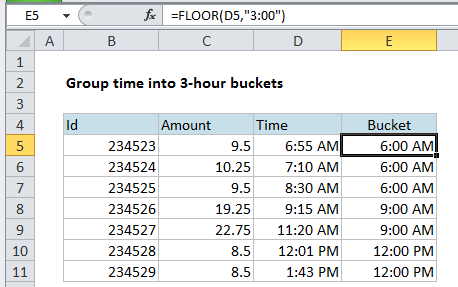Group times into 3 hour buckets in Excel

This tutorial shows how to Group times into 3 hour buckets in Excel using the example below;

Formula

`=FLOOR(time,"3:00")`Explanation

If you need to group times into buckets (i.e. group by 2 hours, group by 3 hours, etc.) you can do so with a rounding function called FLOOR.

In the example shown, we have a number of transactions, each with a time stamp. Let’s say you want to group these transactions into buckets of 3 hours.

That means you would ultimately have buckets like the following:

12:00 AM-3:00 AM
3:00 AM-6:00 AM
6:00 AM-9:00 AM
9:00 AM-12:00 PM

So, for example, a time of 2:30 AM, needs to go into the 12:00 AM – 3:00 AM bucket. A time of 8:45 AM needs to go into the 6:00 AM-9:00 AM bucket, and so on.

If you think about it, one way to do this is to round each time until it fits into the right bucket. However, unlike normal rounding, where we might round to the nearest multiple, in this case, we want to round down to the nearest multiple, starting at midnight.

Because times are just decimal numbers, you can easily do this with the FLOOR function, which rounds down to a multiple that you supply (FLOOR calls the argument that represents multiple “significance”). Even better, FLOOR understands how to round time.

In the example shown, the formula in E5 is:

`=FLOOR(D5,"3:00")`

FLOOR knows how to read time, so it interprets 3:00 as it’s decimal equivalent, 0.125. It then simple rounds down each time to the nearest multiple of 0.125

You can use this same approach to group times into any standard bucket that you like.